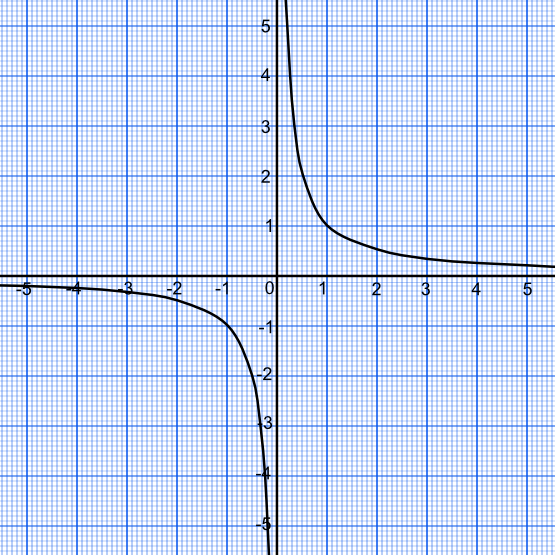Graphing Reciprocal Functions

# Graphing Reciprocal Functions

GCSE(F) GCSE(H)

A reciprocal function, such as y = frac(1)(x), creates two curves on the graph in opposite quadrants. There are values on the graph which cannot be found: x = 0 is a division by zero; and y = 0 requires x to be equal to infinity. The values of x = 0 and y = 0 (in this example) are known as the asymptotes: these are the lines that the curves tend towards, but never touch.## Examples

1. The function y = 3 + frac(1)(x) has been plotted on the graph below. What are the asymptotes for this graph?Answer: x = 0 and y = 3

The value y = 3 requires x to be set to infinity. The value x = 0 is a division by zero.

2. Using the graph, above, estimate the value of y when x = -1.

Check the answer by substituting into the equation: y = 3 +frac(1)(-1) = 2.